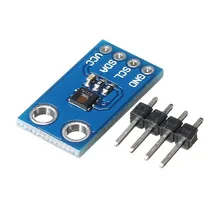# Arduino Uno and HDC1080 humidity and temperature sensor

The HDC1080 is a digital humidity sensor with integrated temperature sensor that provides excellent measurement accuracy at very low power. The HDC1080 operates over a wide supply range, and is a low cost, low power alternative to competitive solutions in a wide range of common applications. The humidity and temperature sensors are factory calibrated.Features
Relative Humidity Accuracy ±2% (typical)
Temperature Accuracy ±0.2°C (typical)
Excellent Stability at High Humidity
14 Bit Measurement Resolution
100 nA Sleep Mode Current

Connection

This was connected an Arduino Uno

 Arduino connection Module connection 3v3 3v3 GND GND SDA – A4 SDA SCL – A5 SCL

Code

You will need to download the following library and install it from  https://github.com/closedcube/ClosedCube_HDC1080_Arduino , this is the default example

 Source code```#include <Wire.h>
#include "ClosedCube_HDC1080.h"

ClosedCube_HDC1080 hdc1080;

void setup()
{
Serial.begin(9600);
Serial.println("ClosedCube HDC1080 Arduino Test");

// Default settings:
// - Heater off
// - 14 bit Temperature and Humidity Measurement Resolutions
hdc1080.begin(0x40);

Serial.print("Manufacturer ID=0x");
Serial.println(hdc1080.readManufacturerId(), HEX); // 0x5449 ID of Texas Instruments
Serial.print("Device ID=0x");
Serial.println(hdc1080.readDeviceId(), HEX); // 0x1050 ID of the device

printSerialNumber();

}

void loop()
{
Serial.print("T=");
Serial.print("C, RH=");
Serial.println("%");
delay(3000);
}

void printSerialNumber() {
Serial.print("Device Serial Number=");
HDC1080_SerialNumber sernum = hdc1080.readSerialNumber();
char format;
sprintf(format, "%02X-%04X-%04X", sernum.serialFirst, sernum.serialMid, sernum.serialLast);
Serial.println(format);
}```

Output

Open the serial monitor window and you should expect to see something like this

T=21.75C, RH=28.00%
T=21.60C, RH=28.21%
T=25.36C, RH=32.17%
T=27.87C, RH=43.27%
T=27.42C, RH=40.23%
T=26.90C, RH=32.15%
T=26.41C, RH=27.84%
T=26.00C, RH=25.77%
T=25.59C, RH=24.80%
T=25.15C, RH=24.43%
T=24.81C, RH=24.34%
T=24.45C, RH=24.47%
T=24.13C, RH=24.68%
T=23.82C, RH=24.89%
T=23.55C, RH=25.30%
T=23.26C, RH=25.72%
T=23.01C, RH=26.14%
T=22.71C, RH=26.64%
T=22.49C, RH=26.96%
T=22.28C, RH=27.27%